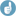## total (pooled) variance: examples [Power / Sample Size]

Dear BEQool, well done!❝ So it seems that the denominator is affected by whether the model is complete or incomplete.

Thank you for correcting me. I didn’t remember it correctly. I was sure about denominator equal to 3 in “All at Once” approach (i.e. one ANOVA). The another (better) approach I didn’t use for past several years and I forgot the details like the denominator used. Sorry for the confusion. So it seems the denominator is equal to the number of treatments in evaluation.Btw. total (pooled) CV in 2x2x2 can also be obtained by fitting the model with factors Formulations, Periods, Sequences (i.e. without Subjects(Sequences)). Then use MSE in equation:
CV=sqrt(e^(MSE)-1) (multiplicative model with residual variance sigma^2 which is estimated by MSE from ANOVA)
For parallel design, it's trivial and only the total (pooled) CV can be obtained.
For higher-order designs with more than two formulations I never tried it but it could be another option for recalculation.

❝ ... it could happen with real-world data as well?

Sometimes (not often) it happens. It was discussed e.g. in this thread.Ing. Helmut Schütz